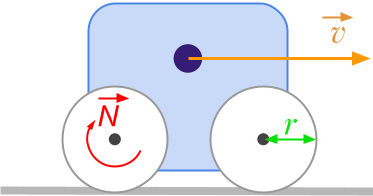Question #102

How to convert revolutions per minute [rpm] to meters per second [m/s]The formula to convert angular velocity expressed in revolutions per minute [﻿﻿ ] to linear speed expressed in meters per second [﻿﻿ ] is given by:

﻿﻿

With the units:

﻿﻿

﻿﻿

where:

• ﻿﻿ is the linear velocity expressed in ﻿﻿
• ﻿﻿ is the angular velocity expressed in ﻿﻿
• ﻿﻿ is the radius expressed in ﻿﻿
11 events in history

Icons proudly provided by Friconix.